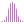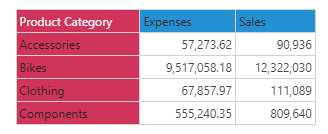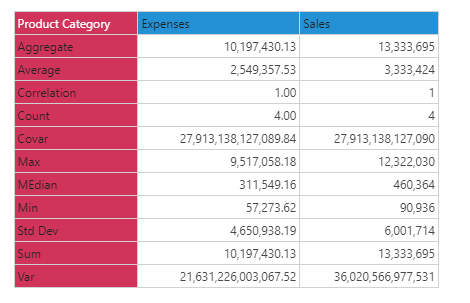#Median (PQL)

Returns the median amount amongst elements in the specified list.

#### Syntax

Median( <List> , OPTIONAL )

* Click on the function's arguments above for more details on the input values.

• List parameter should be a list or set of elements from an existing hierarchy in the model.
• The data point or tuple is optional, and describes the values used to generate the median. If not supplied, the default measure or the query context will determine this value instead.
• For details on how to employ and use this function see the semantic calculation overview.
##### Different Function types
• This function is like the MDX median function; it calculates the median across a list of elements in a hierarchy or attribute.
• If, instead, you wish to change the aggregate of a numeric column in the database, use this function
• To use a simple median mathematical operation on a list of numbers, use the Common library function.

Also see the Percentile function.

#### Examples

This example returns the median expenses across the product category in the product table/ dimension, using the sample demo model:

Median ( {AllMembers([products].[Product Category])}, ([measures].[data Expenses]) )

Below, using the sample data of the 4 product categories we can use the above formula to calculate the median. The list contains 4 values; the median, or middle value, will need to be resolved using interpolation between the 2 inner values (67,857 and 555,240), producing 311,549: